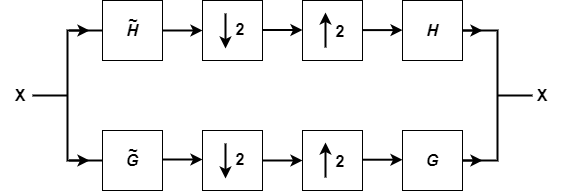# isorthwfb

Determine if filter bank is orthogonal wavelet filter bank

Since R2022b

## Syntax

``tf = isorthwfb(Lo)``
``tf = isorthwfb(Lo,Hi)``
``tf = isorthwfb(___,Tolerance=tol)``
``[tf,checks] = isorthwfb(___)``

## Description

````tf = isorthwfb(Lo)` returns true if the two-channel filter bank formed from the lowpass (scaling) filter `Lo` satisfies the necessary and sufficient conditions to be a two-channel orthonormal perfect reconstruction (PR) wavelet filter bank. `isorthwfb` forms the highpass (wavelet) filter using the `qmf` function: `Hi = qmf(Lo)`. For a list of the necessary and sufficient conditions that the lowpass and highpass filters must satisfy, see Orthonormal Perfect Reconstruction Wavelet Filter Bank.```
````tf = isorthwfb(Lo,Hi)` uses the highpass (wavelet) filter `Hi` to determine whether `Lo` and `Hi` jointly satisfy the necessary and sufficient conditions to be a two-channel orthonormal PR wavelet filter bank.`isorthwfb` assumes that `Lo` and `Hi` form an orthogonal quadrature mirror filter pair. To return accurate results, ensure that you provide either both analysis filters or both synthesis filters.```
````tf = isorthwfb(___,Tolerance=tol)` uses the positive real scalar tolerance `tol` to determine whether the filters satisfy the necessary and sufficient conditions to be a two-channel orthonormal PR wavelet filter bank.```

example

````[tf,checks] = isorthwfb(___)` returns a table with all orthogonality checks.```

## Examples

collapse all

Check the orthogonality conditions for the lowpass (scaling) filter associated with Daubechies least-asymmetric wavelet of order 5. Confirm all the checks pass.

```scalf = symwavf("sym5"); [tf,checks] = isorthwfb(scalf)```
```tf = logical 1 ```
```checks=7×3 table Pass-Fail Maximum Error Test Tolerance _________ _____________ ______________ Equal-length filters pass 0 0 Even-length filters pass 0 0 Unit-norm filters pass 8.6264e-14 1.4901e-08 Filter sums pass 3.3374e-12 1.4901e-08 Even and odd downsampled sums pass 1.669e-12 1.4901e-08 Zero autocorrelation at even lags pass 1.2896e-13 1.4901e-08 Zero crosscorrelation at even lags pass 1.1345e-17 1.4901e-08 ```

Obtain the lowpass and highpass synthesis filters associated with the Coiflet of order 5. Confirm the two-channel filter bank formed from the filter pair satisfies the necessary and sufficient conditions to be a two-channel orthonormal PR wavelet filter bank.

```[~,~,LoR,HiR] = wfilters("coif5"); [tf2,checks2] = isorthwfb(LoR,HiR)```
```tf2 = logical 1 ```
```checks2=7×3 table Pass-Fail Maximum Error Test Tolerance _________ _____________ ______________ Equal-length filters pass 0 0 Even-length filters pass 0 0 Unit-norm filters pass 1.0399e-10 1.4901e-08 Filter sums pass 4.2426e-10 1.4901e-08 Even and odd downsampled sums pass 2.1213e-10 1.4901e-08 Zero autocorrelation at even lags pass 4.1627e-09 1.4901e-08 Zero crosscorrelation at even lags pass 3.5434e-19 1.4901e-08 ```

## Input Arguments

collapse all

Lowpass (scaling) filter, specified as a real-valued vector. `Lo` must have an even number of samples. `Lo` should sum to 1 with an L2 norm of 1/√2, or sum to √2 with an L2 norm of 1.

Example: `Lo = dbwavf("db4")`

Data Types: `single` | `double`

Highpass (wavelet) filter, specified as a real-valued vector. `Hi` and `Lo` must have the same number of samples and be even-length vectors.

Example: `Hi = qmf(Lo)`, where ```Lo = dbwavf("db6")```.

Data Types: `single` | `double`

Tolerance used in filter bank checks, specified as a positive real scalar.

## Output Arguments

collapse all

True or false result, returned as a `1` or `0` of data type `logical`. The `isorthwfb` function returns a `1` if the filters satisfy all the conditions listed in Orthonormal Perfect Reconstruction Wavelet Filter Bank within the specified tolerance.

Orthogonality checks, returned as a table. The table shows `pass` or `fail` for each check as well as the maximum error and specified test tolerance where applicable. A test tolerance of `0` indicates that the check is a logical pass or fail.

collapse all

### Orthonormal Perfect Reconstruction Wavelet Filter Bank

A lowpass filter G and highpass filter H form an orthonormal perfect reconstruction (PR) wavelet filter bank if the filters satisfy certain conditions. The lowpass and highpass analysis filters $\stackrel{˜}{G}$ and $\stackrel{˜}{H},$ respectively, are time reverses of the lowpass and highpass synthesis filters $G$ and $H,$ respectively. This is a diagrammatic representation of the two-channel filter bank.The lowpass and highpass filters form an orthonormal PR wavelet filter bank if all of the following conditions are satisfied.

• `Equal-length filters` — The lowpass and highpass filters have an equal number of coefficients.

• `Even-length filters` — The lowpass and highpass filters have an even number of coefficients.

• `Unit-norm filters` — The L2 norm of each filter is equal to 1.

• `Filter sums` — The sum of the lowpass filter coefficients equals √2, and the sum of the highpass filter coefficients equals 0.

• `Even and odd downsampled sums` — The lowpass filter G satisfies the fundamental condition:

`$\sum _{i=1}^{N/2}{g}_{2i}=\sum _{i=1}^{N/2}{g}_{2i-1},$`

where ${g}_{k}$ is the kth filter coefficient of G and N is the length of G. In other words, the sum of the even-indexed filter coefficients and the sum of the odd-indexed coefficients both equal 1/√2.

• `Zero autocorrelation at even lags` — The autocorrelation of the lowpass and highpass filters at all even nonzero lags equals 0.

• `Zero crosscorrelation at even lags` — The cross-correlation of the lowpass and highpass filters at all even lags equals 0.

## Algorithms

Before performing the orthogonality checks, the `isorthwfb` function normalizes the lowpass filter so its coefficients sum to √2.

 Strang, Gilbert, and Truong Nguyen. Wavelets and Filter Banks. Rev. ed. Wellesley, Mass: Wellesley-Cambridge Press, 1997.

 Burrus, C. S., Ramesh A. Gopinath, and Haitao Guo. Introduction to Wavelets and Wavelet Transforms: A Primer. Upper Saddle River, N.J: Prentice Hall, 1998.